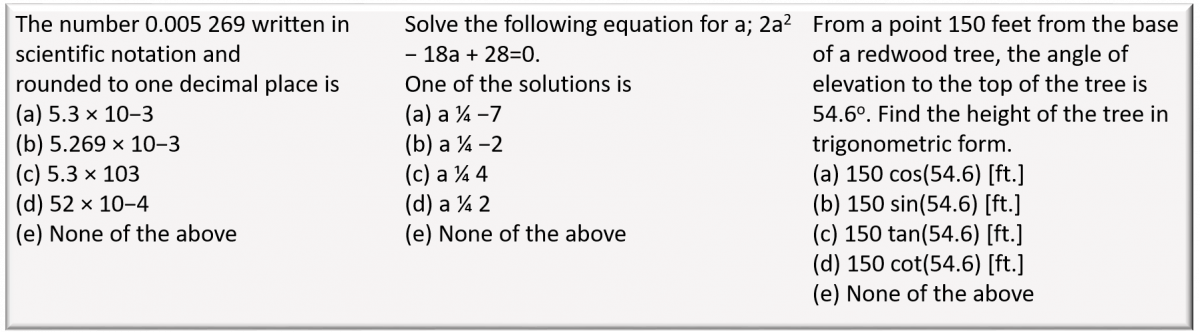# Can Math Tutorials Improve Physics Grades?

Paper: Math remediation intervention for student success in the algebra-based introductory physics course
Authors: Rebecca L. Forrest, Donna W. Stokes, Andrea B. Burridge, Carol D. Voight
First author institute:  University of Houston
Journal: Physical Review Physics Education Research 13 020137 (2017)

Anyone who has taken a physics course can probably tell you that mathematics is an important part of the class. However, math is more than just a tool to do physics; it is essential to one’s success in physics. Many studies have found that math skills and math preparation in high school are related to success in undergraduate, introductory physics courses. Further, scores on diagnostic math tests are correlated with final grades in physics courses. These findings suggest that improving students’ math skills could result in better grades in physics courses, which is the subject of today’s paper.

An unnamed university noticed that only 65% of its students passed its introductory algebra-based physics course, which was much lower than the passing rate of other introductory science courses. These introductory physics courses already included physics education research inspired reforms such as Peer Instruction, Just in Time Teaching, warm up assignments, and Interactive Lecture Demonstrations, but despite positive feedback, had not significantly increased the passing rate of the course. Inspired by previous results linking math skills and physics grades, the authors of today’s paper wanted to determine if improving students’ math skills would increase their chances of passing the introductory physics course.

All students who enroll in the introductory physics course take a 20-item mathematics diagnostic test at the beginning of the course, which counted for a small portion of their final grade. Sample problems from the diagnostic are shown in figure 1.

Since the diagnostic test had been in place for many years before the start of the study, the physics department had determined that a 70% on the diagnostic test signaled the student had the necessary math preparation to pass the class and below a 50% signaled the student needed to significantly improve their math skills in order to do well in the course. For the purpose of this study however, the researchers classified any student who answered less than 65% of the questions correctly “at risk” for not passing the course. Of the 643 students who completed the diagnostic test (and received a final grade in the course), 231 students were deemed “at risk.” The researchers then selected three of the six sections of the course to implement a tutorial designed to improve the students’ math skills. The tutorial was created from questions available in MyMathTest and consisted of four parts. First, the student completed a subset of problems similar to those that were on the diagnostic exam. Sample problems appear in figure 2.

The MyMathTest program then identified the areas in which the student was weakest and created additional problems for the student to work through. This process was repeated three times. After the third time, the student completed a final test. All students in the three sections with the tutorial implemented were able to complete the tutorial but “at risk” students were given a small grade incentive to complete the tutorial. Of the 125 “at risk” students who were given the opportunity to complete the tutorial, 61 of them did.

The researchers found that 83.6% of the students who completed the tutorial passed the course while only 59.4% of the “at risk” students who did not complete the tutorial passed the course. The researchers found that women were more likely than men were to complete the tutorial, but students of different races and ethnicities completed the tutorial at similar rates.

Next, the researchers wanted to determine how completing the tutorial affected the odds of passing the course. To do so, the researchers used generalized estimating equations logistic regression, which tries to predict whether the student passed or failed the course based on a variety of factors including completing the tutorial. The researchers found that students who completed the tutorial had a 4.24 times larger odds of passing the course than students who did not complete the tutorial.

After determining that completing the tutorial increased the odds a student would pass the class, the researchers wanted to determine how much their grade increased as a result of the tutorial. To do this, the researchers used generalized estimating equations linear regression and tried to predict the actual numeric grade of the student from various factors including completing the tutorial. The researchers found that completing the tutorial increased the student’s grade by roughly 11 points, or one letter grade.

While these results seem to suggest that the tutorial is very effective in improving the number of students passing the course, the researchers still weren’t sure. What if the students who had completed the tutorial were simply more motivated or better prepared academically in other areas and that is why the tutorial seemed to work. To check this hypothesis, the researchers compared the SAT scores (which have been shown to be related to academic preparation and certain motivational traits) of those who completed the tutorial and those who didn’t and found no statistical difference. This result suggests that the tutorial, and not some difference in the students, accounted for the nearly one letter grade increase in the final grades.

So what can we take away from this study? First, it appears that improving the math skills of students who are “at risk” for failing the course significantly decreases the odds of them failing the course. Second, the intervention doesn’t require the instructor’s involvement and can be completed outside of the classroom, making it practical for large enrollment classes. As the authors note, African American students were at the greatest risk for failing the course, so implementing small interventions such as the one described here could be essential for increasing the number of underrepresented minorities in physics.